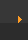## Stoichiometry: Mole to Mole Conversions

 There are two ways to solve problems where you are given a balanced equation and asked to predict how many moles of a substance are formed (or used). The first method (preferred by scientists) uses a mole to mole ratio from the balanced equation to cancel out units and solve the problem. This is similar to how we have approached problems in the other sections. The second method is easier but you have to be very careful to make sure you have the correct units. Try this method if you're struggling with the first method. Try to solve the problem yourself, then watch the video. Then try it again. Just watching won't improve your ability to solve problems—you need to try it yourself. How many moles of H2O are produced when 3.2 mol of O2 are reacted with H2? Method One Method Two How many moles of carbon monoxide (CO) will be produced when 2.71 moles of carbon (C) are reacted with SiO2? (Try, then watch video.) Mole to Mole Conversions: Method One Mole to Mole Conversions: Method Two If you start with 4.2 moles of Fe, how many moles of FeCl2 will be produced? (Try, then watch video.) Method One Method Two